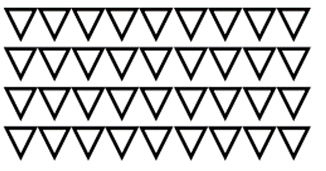Mathematics
Easy

Question

# Choose the correct multiplication sentence this array show.## 4 × 9    4 × 10 = 40    4 × 10    4 × 9 = 36Hint:

## The correct answer is: 4 × 9 = 36

### In the question there is an array using which we have to find the multiplicative expression of that array.The number of rows = 4The number of columns = 9The multiplicative expression= 4 × 9 = 36.So, the multiplicative expression of the given array will be 4 × 9 = 36.Therefore, the correct option is d, i.e., 4 × 9 = 36.

For simple multiplication we have to remember the multiplication table of one-digit numbers and for two-digit number we can find it using the multiplication table of one-digit numbers. Here, we have to find the product of one digit number.

### Related Questions to study#### With Turito Foundation.#### Get an Expert Advice From Turito.